A voltmeter has resistance of 2000 ohms and it can measure upto 2V. If we want to increase its range to 10 V, then the required resistance in series will be

(1) 2000 Ω

(2) 4000 Ω

(3) 6000 Ω

(4) 8000 Ω

High Yielding Test Series + Question Bank - NEET 2020

Difficulty Level:

The ammeter A reads 2 A and the voltmeter V reads 20 V. the value of resistance R is (Assuming finite resistance's of ammeter and voltmeter)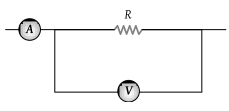(1) Exactly 10 ohm

(2) Less than 10 ohm

(3) More than 10 ohm

(4) We cannot definitely say

High Yielding Test Series + Question Bank - NEET 2020

Difficulty Level:

The resistance of a galvanometer coil is R. What is the shunt resistance required to convert it into an ammeter of range 4 times

(1) $\frac{R}{5}$

(2) $\frac{R}{4}$

(3) $\frac{R}{3}$

(4) 4 R

High Yielding Test Series + Question Bank - NEET 2020

Difficulty Level:

In the given figure, battery E is balanced on 55 cm length of potentiometer wire but when a resistance of 10 Ω is connected in parallel with the battery then it balances on 50 cm length of the potentiometer wire then internal resistance r of the battery is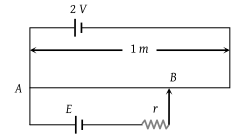(1) 1 Ω

(2) 3 Ω

(3) 10 Ω

(4) 5 Ω

High Yielding Test Series + Question Bank - NEET 2020

Difficulty Level:

A galvanometer has a resistance of 25 ohm and a maximum of 0.01 A current can be passed through it. In order to change it into an ammeter of range 10 A, the shunt resistance required is

(1) 5/999 ohm

(2) 10/999 ohm

(3) 20/999 ohm

(4) 25/999 ohm

High Yielding Test Series + Question Bank - NEET 2020

Difficulty Level:

In the circuit shown, a meter bridge is in its balanced state. The meter bridge wire has a resistance 0.1 ohm/cm. The value of unknown resistance X and the current drawn from the battery of negligible resistance is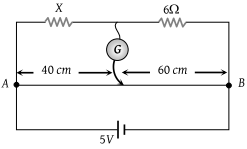(1) 6 Ω, 5 amp

(2) 10 Ω, 0.1 amp

(3) 4 Ω, 1.0 amp

(4) 12 Ω, 0.5 amp

Concept Questions :-

Meter bridge and potentiometer
High Yielding Test Series + Question Bank - NEET 2020

Difficulty Level:

AB is a potentiometer wire of length 100 cm and its resistance is 10 ohms. It is connected in series with a resistance R = 40 ohms and a battery of e.m.f. 2 V and negligible internal resistance. If a source of unknown e.m.f. E is balanced by 40 cm length of the potentiometer wire, the value of E is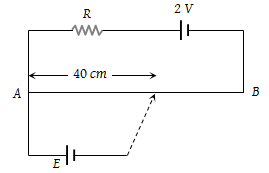(1) 0.8 V

(2) 1.6 V

(3) 0.08 V

(4) 0.16 V

Concept Questions :-

Meter bridge and potentiometer
High Yielding Test Series + Question Bank - NEET 2020

Difficulty Level:

The measurement of the voltmeter in the following circuit is :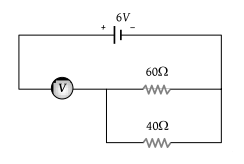(1) 2.4 V

(2) 3.4 V

(3) 4.0 V

(4) 6.0 V

Concept Questions :-

Kirchoff's voltage law
High Yielding Test Series + Question Bank - NEET 2020

Difficulty Level:

The potential difference across the 100Ω resistance in the following circuit is measured by a voltmeter of 900 Ω resistance. The percentage error made in reading the potential difference is :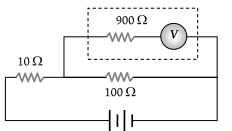(1) $\frac{10}{9}$

(2) 0.1

(3) 1.0

(4) 10.0

Concept Questions :-

Combination of resistors
High Yielding Test Series + Question Bank - NEET 2020

Difficulty Level:

If the ammeter in the given circuit reads 2 A, the resistance R is :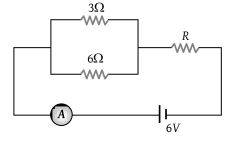(1) 1 ohm

(2) 2 ohm

(3) 3 ohm

(4) 4 ohm

Concept Questions :-

Kirchoff's voltage law